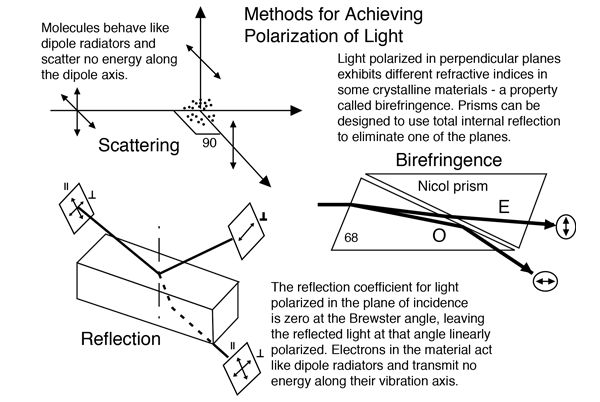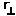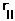Index

Polarization concepts

 HyperPhysics***** Light and Vision R Nave
Go Back

# Polarization by ReflectionCalculation Derivation of Brewster's angle Methods of achieving polarization
Index

Polarization concepts

 HyperPhysics***** Light and Vision R Nave
Go Back

# Reflected Intensity

For an incident angle of
α = °
and an index
n = ,
the transmitted angle is
β = °. The reflection coefficients are.==

The overall reflected intensity is % of the incident and % of that is in the perpendicular plane. The Brewster angle is .Note: the reflection coefficients used here are the intensities and not the amplitudes as used in the usual presentation of the Fresnel equations. That is, these reflection coefficients are the square of those in the Fresnel expressions.

 Polarization by reflection Snell's law
Index

Polarization concepts

 HyperPhysics***** Light and Vision R Nave
Go Back

# Polarization by ScatteringThe scattering of light off air molecules produces linearly polarized light in the plane perpendicular to the incident light. The scatterers can be visualized as tiny antennae which radiate perpendicular to their line of oscillation. If the charges in a molecule are oscillating along the y-axis, it will not radiate along the y-axis. Therefore, at 90° away from the beam direction, the scattered light is linearly polarized. This causes the light which undergoes Rayleigh scattering from the blue sky to be partially polarized.
 Methods of achieving polarization
Index

Polarization concepts

 HyperPhysics***** Light and Vision R Nave
Go Back